Chapter 3 Part 2 - Banking

Economics Class 12
Macroeconomics

##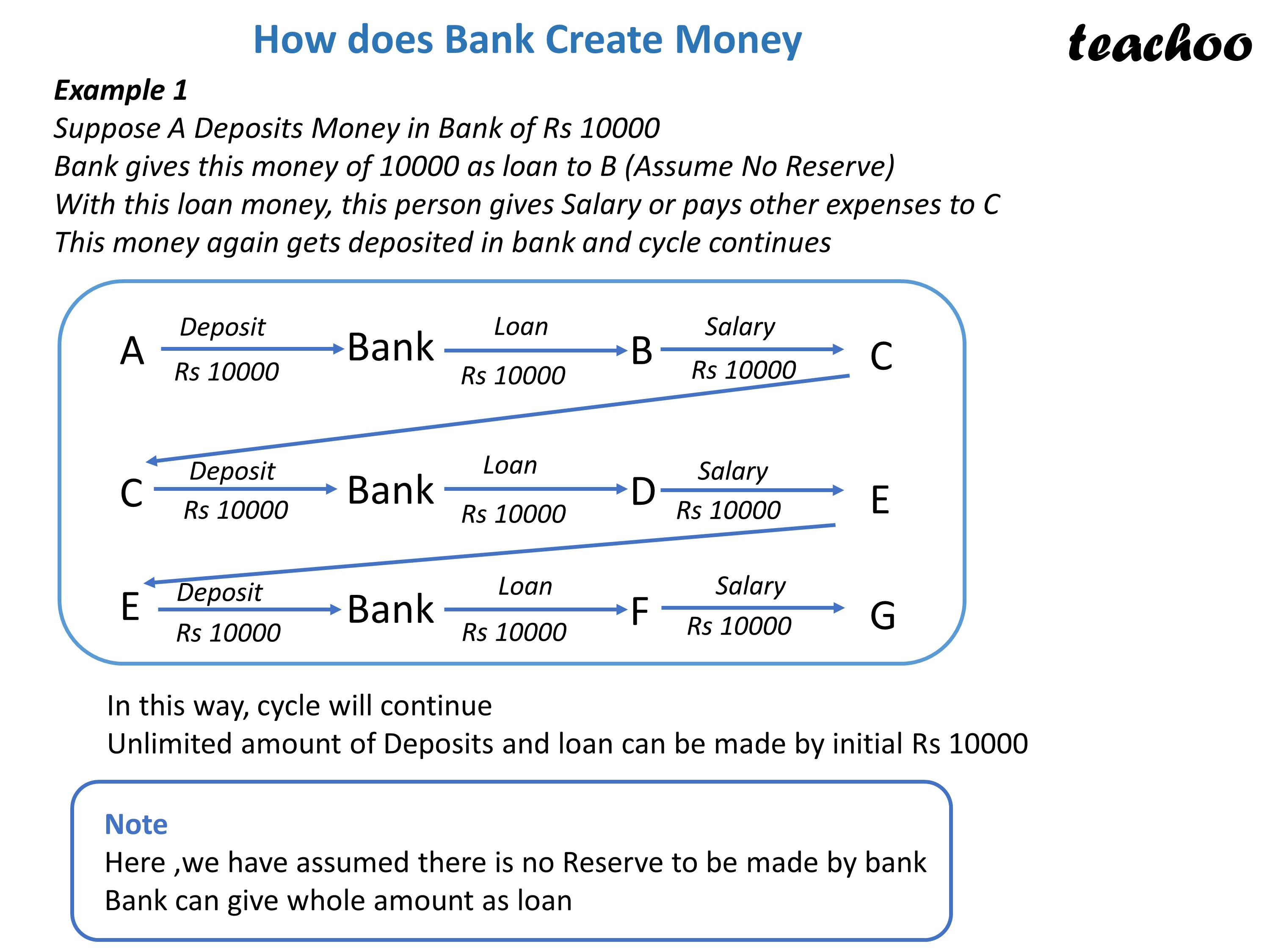googletag.cmd.push(function() { googletag.display('div-gpt-ad-1669298377854-0'); });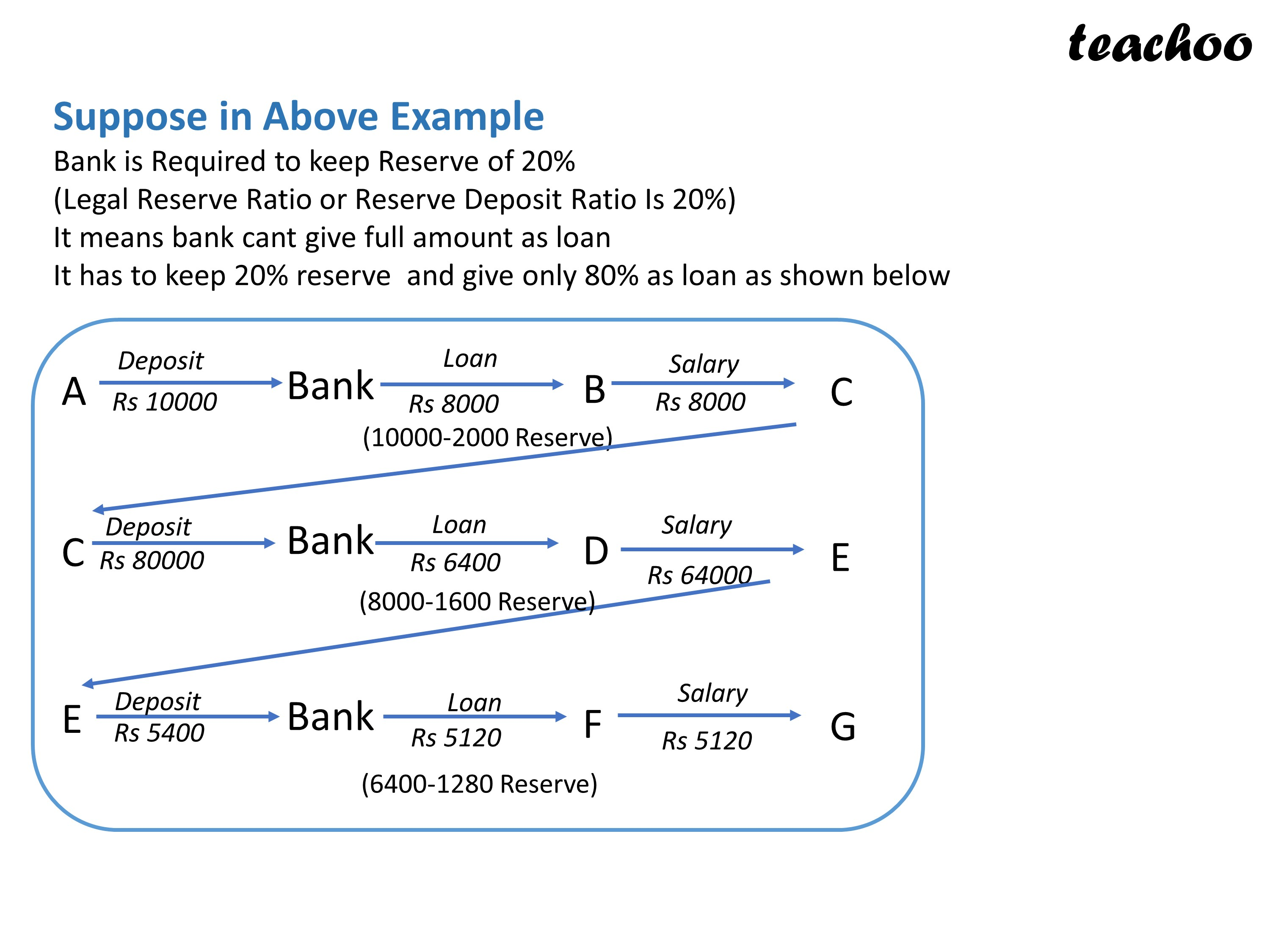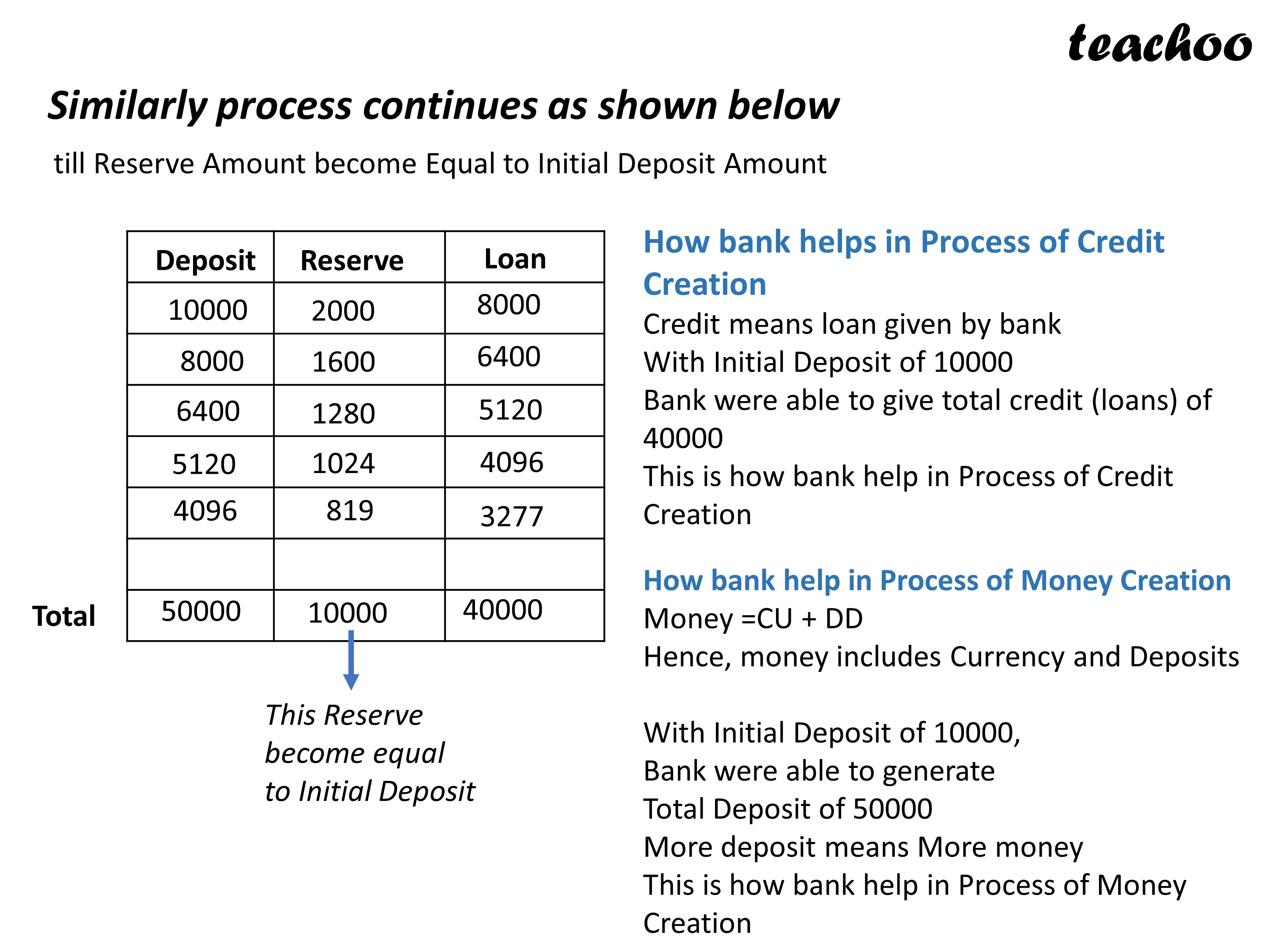## Explain how banks help in the Process of Money Creation and Credit Creation

We know that Banks take Deposits from Public and provide loans or credit to other people with that money

Suppose Banks Receive Deposits of Rs 1,00,000

They cannot give loan of whole Rs 1,00,000

They have to keep a certain amount as Reserve (called Reserve Deposit Ratio or Legal Reserve Ratio)

Suppose Legal Reserve Ratio is 20%

It means Banks have to keep 20,000 with them as Reserve

They can give loan of balance 80,000

Now when bank gives Balance Rs 80,000 as loan

Person who receives this loan money makes a number of expenses with this money

Lets assume He makes expenses of Rs 80,000 with this money as Salary to employee

This Employee will again deposit this Rs 80,000 with bank

Banks will retain Reserve of 20% (Rs80,000*20% = Rs16,000)

They will give loan of balance amount Rs 80,000 - 16,000 = Rs 64,000

Similarly the process continues as shown below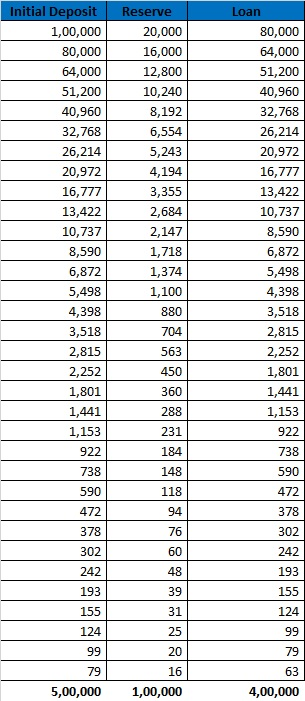## Note

More we go down ,the amount of reserves will diminish

It will soon come a point where Total Reserves will be equal to Initial amount deposited

Initial Amt deposited = Total Reserves = Rs 1,00,000

At this Stage, Total Deposits will be Rs 5,00,000

and Total loans will be Rs 4,00,000

Hence, we can Say that

With Initial Deposits of Rs 1,00,000

Banks are able to generate Deposits of Rs 5,00,000 and Credit of Rs 4,00,000

## What is Money Multiplier?

It is the multiple by which initial deposits increase

It is the Ratio of Deposits Created over Initial Deposits

Money Multiplier = Deposits Created/Initial Deposits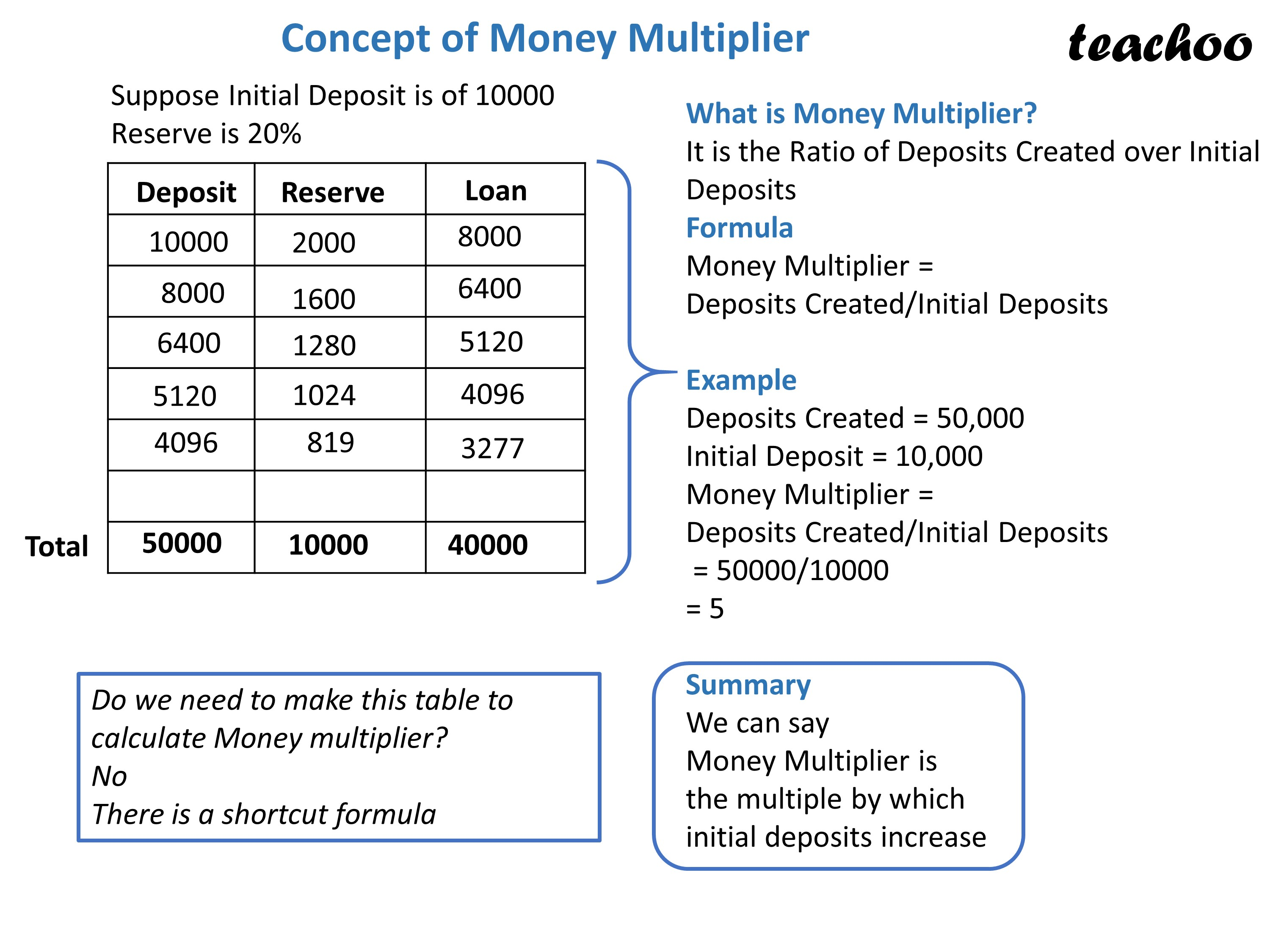Formula for Calculating Money Multiplier

(Without making Table)

Money Multiplier = 1/Legal Reserve Ratio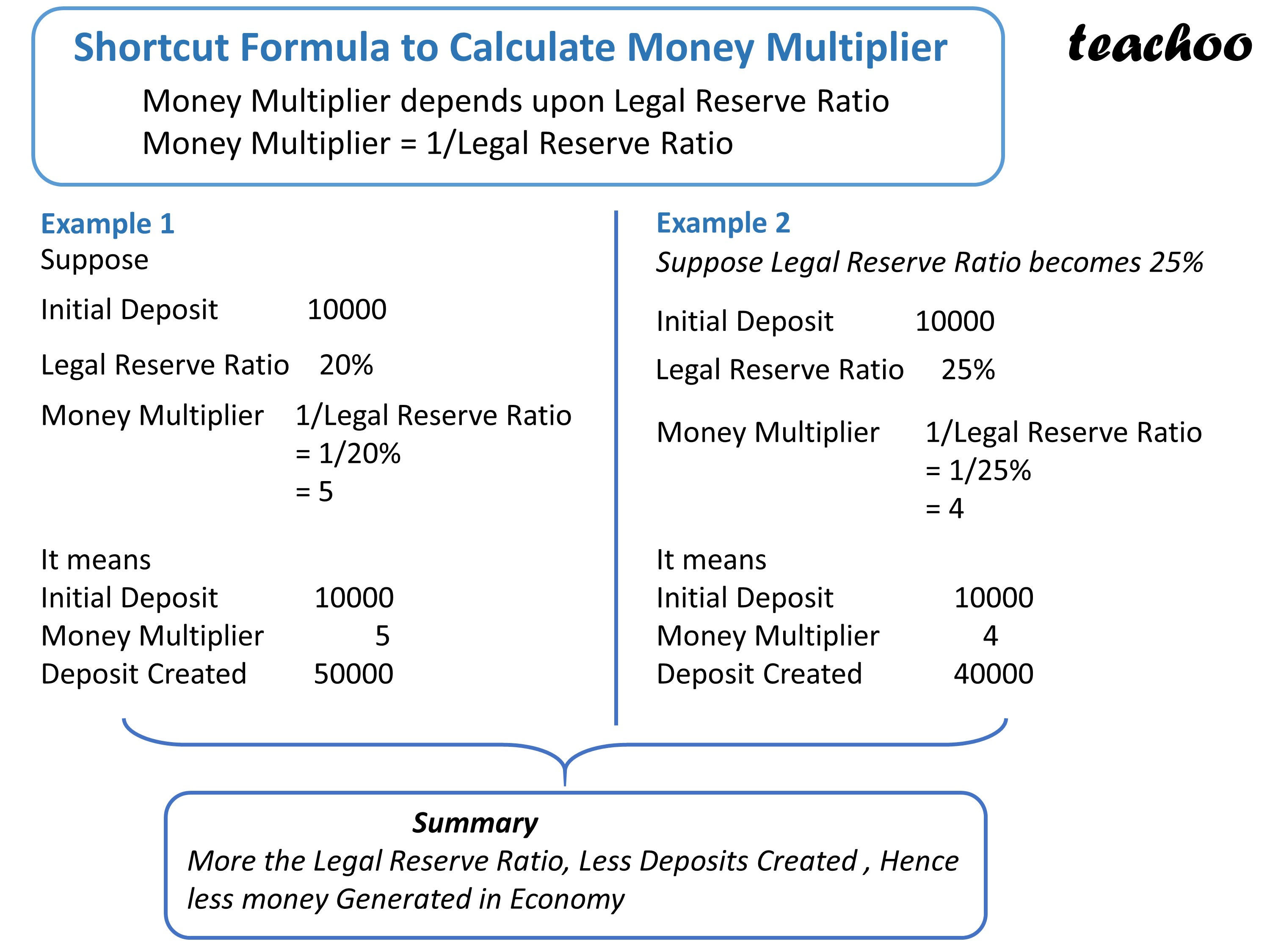### NC113:C198

#### Question 8

What is money multiplier?

What determines the value of this multiplier?

#### Question 10

Do you consider a commercial bank ‘creator of money’ in the economy?

### Other Books

#### Question 1

In the following questions, select the correct answers:

Through the process of _____, commercial banks are able to create credit, which is in far excess of the initial deposits.

b. Money Creation

c. Credit Creation

d. Accepting Deposits

#### Question 2

Banks create credit:

a. Out of nothing

b. On the basis of their securities

c. On the basis of their total assets

d. On the basis of deposits

#### Question 3

If the total deposits created by commercial banks is Rs10,000 crores and LRR is 40%,

then amount of initial deposits will be ____.

a. Rs 2000 crores

b. Rs 3000 crores

c. Rs 4000 crores

d. Rs 14000 crores

#### Question 4

Deposit creation by banks come to an end when:

a. Fresh deposits with banks become zero

b. Legal Reserve Ratio becomes zero

c. Money Multiplier becomes zero

d. Total Cash Reserves becomes equal to Initial Deposits

#### Question 5

The value of multiplier will be ____, when LRR = 20%.

a. 2

b. 3

c. 4

d. 5

Learn in your speed, with individual attention - Teachoo Maths 1-on-1 Class

### Transcript

Shortcut Formula to Calculate Money Multiplier Money Multiplier depends upon Legal Reserve Ratio Money Multiplier = 1/Legal Reserve Ratio Example 1 Initial Deposit 10000 Legal Reserve Ratio 20% Money Multiplier 1/Legal Reserve Ratio = 1/20% = 5 Example 2 Suppose Legal Reserve Ratio becomes 25% Legal Reserve Ratio 25% Initial Deposit 10000 It means Initial Deposit 10000 Money Multiplier 4 Deposit Created 40000 It means Initial Deposit 10000 Money Multiplier 5 Deposit Created 50000 Summary More the Legal Reserve Ratio, Less Deposits Created , Hence less money Generated in Economy Suppose Initial Deposit is of 10000 Reserve is 20% Concept of Money Multiplier What is Money Multiplier? It is the Ratio of Deposits Created over Initial Deposits Formula Money Multiplier = Deposits Created/Initial Deposits Example Deposits Created = 50,000 Initial Deposit = 10,000 Money Multiplier = Deposits Created/Initial Deposits = 50000/10000 = 5 Summary We can say Money Multiplier is the multiple by which initial deposits increase Do we need to make this table to calculate Money multiplier? No There is a shortcut formula Similarly process continues as shown below till Reserve Amount become Equal to Initial Deposit Amount How bank helps in Process of Credit Creation Credit means loan given by bank With Initial Deposit of 10000 Bank were able to give total credit (loans) of 40000 This is how bank help in Process of Credit Creation How bank help in Process of Money Creation Money =CU + DD Hence, money includes Currency and Deposits With Initial Deposit of 10000, Bank were able to generate Total Deposit of 50000 More deposit means More money This is how bank help in Process of Money Creation This Reserve become equal to Initial Deposit Suppose in Above Example Bank is Required to keep Reserve of 20% (Legal Reserve Ratio or Reserve Deposit Ratio Is 20%) It means bank cant give full amount as loan It has to keep 20% reserve and give only 80% as loan as shown below Why is Central Bank called Controller of Credit in Economy Quantitative Measures It is because RBI can Increase or decrease amount of loans given by commercial banks by using Quantitative Measures and Qualitative Measures It refers to measures which control volume of credit Qualitative Measures It refers to measures which control direction of credit Example Moral Suasion (Central Bank may persuade banks to give loan to weaker section of society ) Selective Credit Controls (Central Bank may ask banks not to give loan to particular sector of economy ike Construction Sector) These are Specific in Nature (Affects Particular Sector) It is modern method of Credit Control These are General in Nature (Affects whole economy) It is traditional method of Credit Control